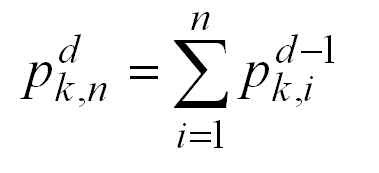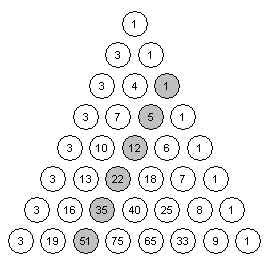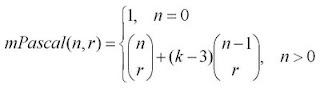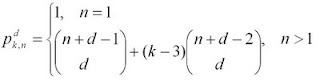## Tuesday, July 22, 2008

### Higher Polygonal Numbers and Pascal's TriangleThe third diagonal column in Pascal's Triangle (r = 2 in the usual way of labeling and numbering) consists of the triangular numbers (1, 3, 6, 10, ...) - numbers that can be arranged in 2-dimensional triangular patterns. The fourth column of Pascal's triangle gives us triangular-based pyramidal numbers (1, 4, 10, 20, ...), built by stacking the triangular numbers. The columns further out give "higher dimensional" triangular numbers that arise from stacking the triangular numbers from the previous dimension.

It is not by coincidence that the triangular and higher-dimensional triangular numbers appear in Pascal's Triangle. If you think about layering of polygonal numbers in terms of equations, you getIn the above equation p^d_(k,n) is the nth k-polygonal number of dimension d. Triangular numbers are the 3-polygonal numbers of dimension 2, square numbers are the 4-polygonal numbers of dimension 2, "square based pyramidal numbers" would be denoted as p^3_(4,n).

From the sum above, you can obtain this equation:Which looks very much like the Pascal Identity C(n,r) = C(n-1,r-1) + C(n-1,r), except for some translation of the variables. To be precise, if we consider the case where k=3 and use r = d and n' = n+d-1 we can translate the triangular numbers into the appropriate positions in Pascal's Triangle.

Along with the definitions for the end columns, the Pascal Identity allows us to generate the whole triangle. This suggests the following strategy for calculating the higher k-Polygonal numbers: create a modified Pascal's Triangle whose first column is equal to k-2 (instead of 1), and whose last column is equal to 1 (as usual). This modified Pascal's Triangle is generated using these initial values and the usual Pascal Identity.

Here is an example with k=5, which sets the first column values equal to 3 (except for the top value, which we keep as 1) and yields the pentagonal numbers (column 3) and the higher pentagonal numbers.The formula for these modified Pascal Triangles is given by this equation:If we apply the change of variables mentioned above, we can obtain this general formula for the higher polygonal numbers in terms of combinations:This formula illustrates how polygonal numbers are built out of triangular numbers. It says that the nth d-dimensional k-polygonal number is equal to the nth d-dimensional triangular number, plus (k-3) copies of the n-1 d-dimensional triangular number. This is a little easier to understand when you forget about the higher-dimensions and look at the regular 2-dimensional polygonal numbers, as described in another post.